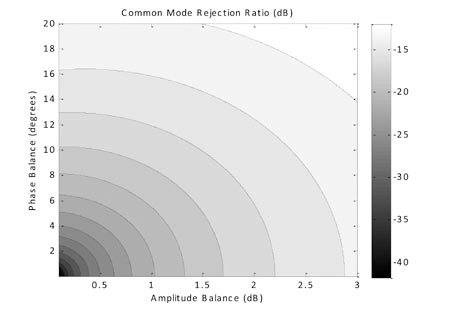# [Tool] A Simple Spreadsheet to Calculate Image Rejection

Make it to the Right and Larger Audience

Blog

# [Tool] A Simple Spreadsheet to Calculate Image Rejection

Here is simple xls to calculate image rejection. Equation is straightforward as$Image Rejection = \frac{1+\gamma^2+2*\gamma*cos(\phi)}{1+\gamma^2-2*\gamma*cos(\phi)}$

where$\gamma$ is amplitude imbalance and$\phi$ is phase imbalance.Senior Engineer at Qualcomm Inc.
Author brief is empty
Groups:

Tags: